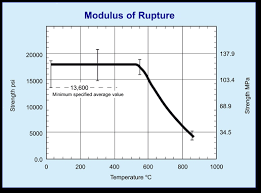## How to Calculate and Solve for Modulus of Rupture | Fracture MechanicsThe image above represents modulus of rupture.

To compute for modulus of rupture, three essential parameters are needed and these parameters are Bending Moment (M), Distance (c) and Moment of Inertia (I).

The formula for calculating modulus of rapture:

σ = Mc / I

Where:

σ = Modulus of Rupture
M = Bending Moment
c = Distance
I = Moment of Inertia

Let’s solve an example;
Find the modulus of rupture when the bending moment is 22, the distance is 10 and the moment of inertia is 14.

This implies that;

M = Bending Moment = 22
c = Distance = 10
I = Moment of Inertia = 14

σ = Mc / I
σ = 22(10) / 14
σ = 220 / 14
σ = 15.71

Therefore, the modulus of rupture is 15.71 Pa.

Calculating the Bending Moment when the Modulus of Rupture, the Distance and the Moment of Inertia is Given.

M = σ x I / c

Where;

M = Bending Moment
σ = Modulus of Rupture
c = Distance
I = Moment of Inertia

Let’s solve an example;
Find the bending moment when the modulus of rupture is 22, the distance is 8 and the moment of inertia is 2.

This implies that;

σ = Modulus of Rupture = 22
c = Distance = 8
I = Moment of Inertia = 2

M = σ x I / c
M = 22 x 2 / 8
M = 44 / 8
M = 5.5

Therefore, the bending moment is 5.5.